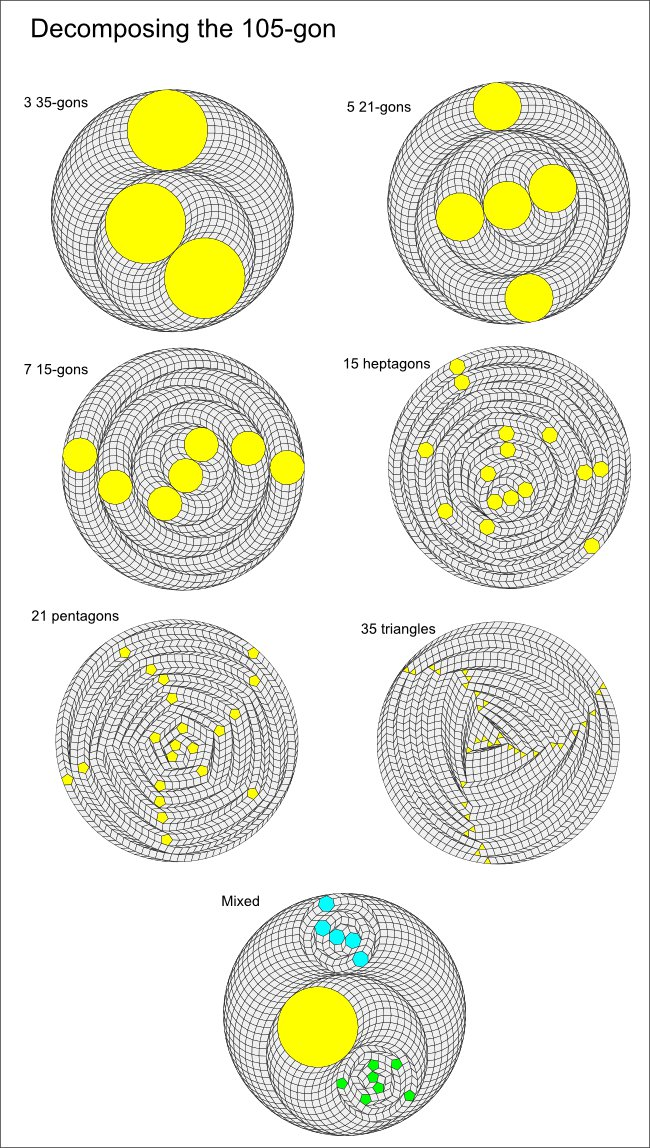# Regular polygon decomposition (odd)

Here's an example for a regular polygon with an odd-number of sides: 105 = 3x5x7It turns out that the decomposition options are more limited if the regular polygon has an odd number of sides:

### Decomposition theorem (odd case)

If a regular polygon has j = mn sides where j is odd with m > 1 and n > 2, then it can be decomposed into rhombs and m n-sided regular polygons.

(Notice that this theorem does not cover the case of decomposition into more than one type of regular polygon. This is often possible as the last example in the above illustration shows where the 105-gon is first decomposed into 3 35-gons, and then two of those 35-gons are further decomposed into 5 heptagons and 7 pentagons respectively.)

In the next sections we'll turn our attention to looking at the algorithm that constructs these decompositions and a proof of the decomposition theorem.